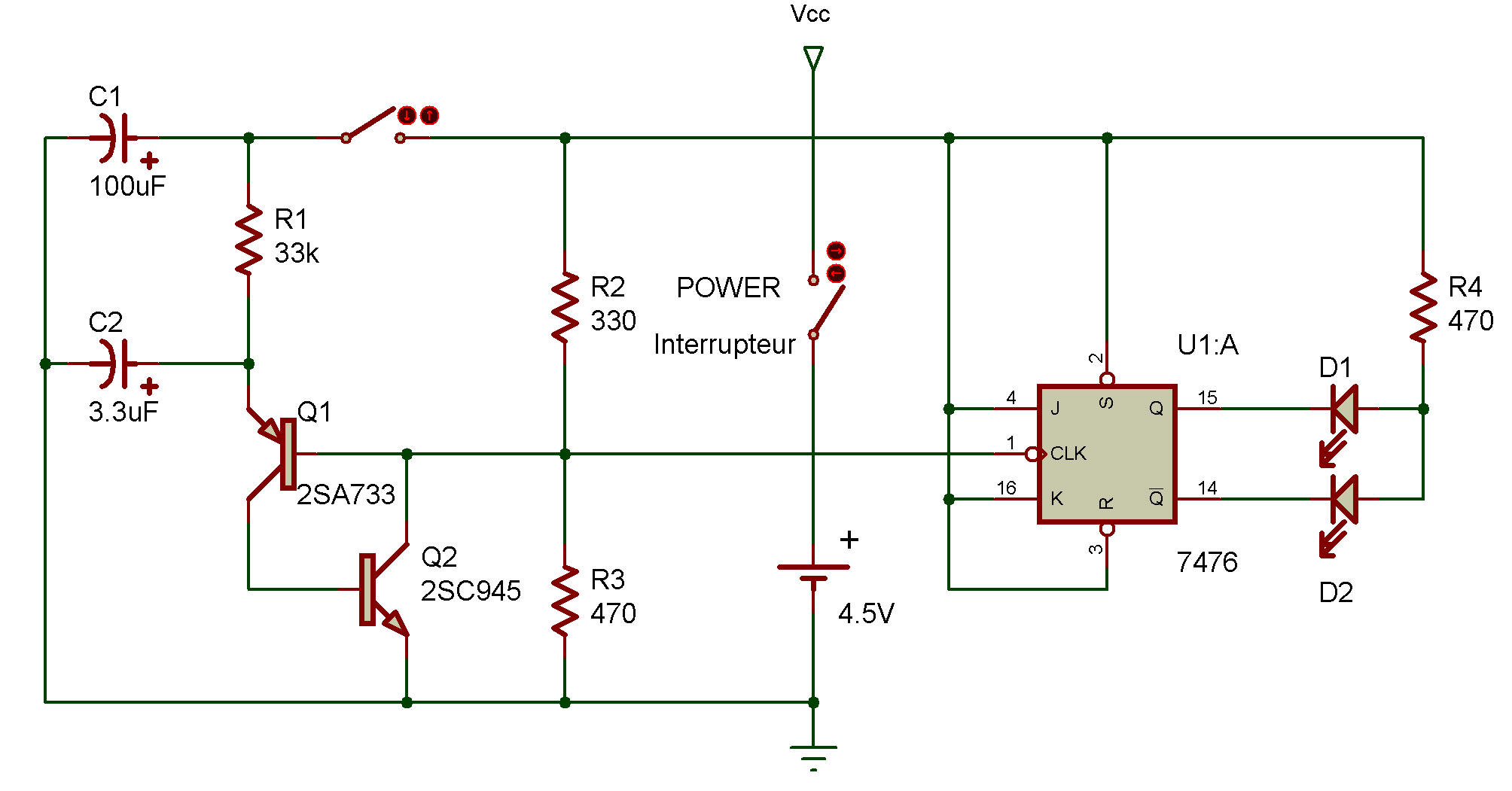Electronic Coin Toss II
Electronic
7476Here's a different version of our last Project Electronic Coin Toss I. If you aren't happy with the decisions the last circuit made, then try this one!

You use this Project almost like Project Electronic Coin Toss I.

Turn the Control Knob to ON. Now press the Key. The two LEDs will take turns lighting and going off. When you release the Key, only one LED will remain lit.

You can see by the schematic that this circuit uses the R-S flip-flop integrated circuit. Look at how the LEDs are connected - one is connected to a point called Q while the other is connected to a point called Q.

Electronics engineers call Q the set output while the Q output is called the reset output. Remember what we mean when we say a digital device is 0 or 1?

With an R-S flip-flop, we say the flip-flop is set if Q is 1 and Q is 0. But if Q is 0 and Q is 1, then the flip-flop is rese t.

Armed with that bit of knowledge, try your hand at this: which LED is lit if the flip-flop is set?

Which one is lit if it's reset?

Can you also figure out how or why the R-S flip-flop sets and resets?

Do you suppose it has anything to do with the connection point marked CK?

Try the same experiment you did with Project Electronic Coin Toss I to see if this is a random circuit.

Is it more or less random than the previous Project?

Or are both the same?
(If you can't decide, try letting one of the two Projects decide for you!)

Recherche personnalisée